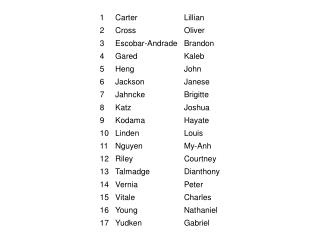DownloadDownload Presentationv / t 2 vx 2 v 2 / t at

# v / t 2 vx 2 v 2 / t at

Download Presentation## v / t 2 vx 2 v 2 / t at

- - - - - - - - - - - - - - - - - - - - - - - - - - - E N D - - - - - - - - - - - - - - - - - - - - - - - - - - -
##### Presentation Transcript

1. Which formula is dimensionally consistent with an expression yielding a value for velocity? (a is acceleration, x is distance, and t is time) • v/t2 • vx2 • v2/t • at

2. If a is acceleration, v is velocity, x is position, and t is time, then which equation is not dimensionally correct? • t = x/v • a = v2/x • v = a/t • t2 = 2x/a

3. When NASA was communicating with astronauts on the moon, the time from sending on the Earth to receiving on the moon was 1.28 s. Find the distance from Earth to the moon. (The speed of radio waves is 3.00  108 m/s.) • 240,000 km • 384,000 km • 480,000 km • 768,000 km

4. A change in a physical quantity w having initial value wi and final value wf is given by which of the following? • wi–wf • wf–wi • (wf + wi)/2 • none of these

5. An object moves 20 m east in 30 s and then returns to its starting point taking an additional 50 s. If west is chosen as the positive direction, what is the sign associated with the average velocity of the object? • + • – • 0 (no sign) • any of these

6. A bird, accelerating from rest at a constant rate, experiences a displacement of 28 m in 11 s. What is the average velocity? • 1.7 m/s • 2.5 m/s • 3.4 m/s • zero

7. Jeff throws a ball straight up. For which situation is the vertical velocity zero? • on the way up • at the top • on the way back down • none of these

8. A railroad train travels forward along a straight track at 80.0 m/s for 1000 m and then travels at 50.0 m/s for the next 1000 m. What is the average velocity? • 65.0 m/s • 61.5 m/s • 63.7 m/s • 70.0 m/s

9. On a position versus time graph, the slope of the straight line joining two points on the plotted curve that are separated in time by the interval ∆t, is which of the following quantities? • average steepness • average velocity • instantaneous velocity • average acceleration

10. An x vs. t graph is drawn for a ball moving in one direction. The graph starts at the origin and at t = 5 s the velocity of the ball is zero. We can be positive that at t = 5 s, • the slope of the curve is non-zero. • the ball has stopped. • the acceleration is constant. • the curve is at x = 0, t = 0.

11. The value of an object’s acceleration may be characterized in equivalent words by which of the following? • displacement • rate of change of displacement • velocity • rate of change of velocity

12. A 50 g ball traveling at 25.0 m/s is bounced off a brick wall and rebounds at 22.0 m/s. A high speed camera records this event. If the ball is in contact with the wall for 3.50 ms, what is the magnitude of the average acceleration of the ball during this time interval? • 13,400 m/s2 • 6,720 m/s2 • 857 m/s2 • 20 m/s2

13. The slope of the acceleration vs. time curve represents: • the velocity. • the rate of change of acceleration. • the rate of change of displacement. • the area under the position vs. time curve.

14. A strobe photograph shows equally spaced images of a car moving along a straight road. If the time intervals between images is constant, which of the following cannot be positive? • the speed of the car • the average velocity of the car • the acceleration of the car • the direction of motion of the car

15. A strobe photograph of a car moving along a straight road shows the interval between each successive image to be diminishing. If the direction of motion of the car is taken as positive, which of the following are negative? • the speed of the car • the average velocity of the car • the average acceleration of the car • all of these

16. A ball is pushed with an initial velocity of 4.0 m/s. The ball rolls down a hill with a constant acceleration of 1.6 m/s2. The ball reaches the bottom of the hill in 8.0 s. What is the ball’s velocity at the bottom of the hill? • 10 m/s • 12 m/s • 16 m/s • 17 m/s

17. A bird, accelerating from rest at a constant rate, experiences a displacement of 28 m in 11 s. What is the final velocity after 11 s? • 1.8 m/s • 3.2 m/s • 5.1 m/s • zero